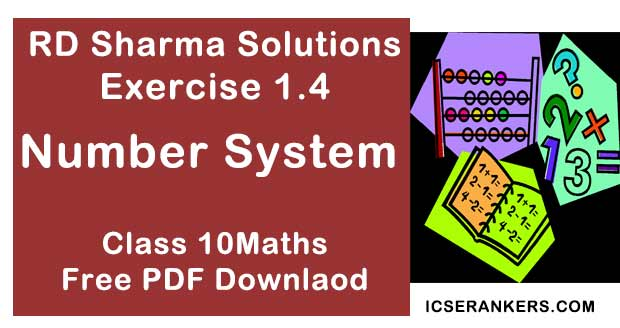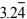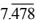# Chapter 1 Number System RD Sharma Solutions Exercise 1.4 Class 9 MathsChapter Name RD Sharma Chapter 1 Number System Exercise 1.4 Book Name RD Sharma Mathematics for Class 10 Other Exercises Exercise 1.1Exercise 1.2Exercise 1.3Exercise 1.5Exercise 1.6 Related Study NCERT Solutions for Class 10 Maths

### Exercise 1.4 Solutions

1. Define an irrational number.

Solution

A number which can neither be expressed as a terminating decimal nor as a repeating decimal is called an irrational number. For example, 1.01001000100001......

2. Explain, how irrational numbers differ from rational numers ?

Solution

A number which can neither be expressed as a terminating decimal nor as a repeating decimal is called an irrational number, for example,and 6.2876 are rational numbers.

3. Examine, whether the following numbers are rational or irrational:
(i) √7
(ii) √4
(iii) 2 + √3
(iv) √3 + √2
(v) √3 + √5
(vi) (√2 – 2)2
(vii) (2 - √2)(2 + √2)
(viii) (√2 + √3)
(ix) √5 - 2
(x) √23
(xi) √225
(xii) 0.3796
(xiii) 7.478478
(xiv) 1.101001000100001.....

Solution

√7 is not a perfect square root, so it is an irrational number.
We have,
√4 = 2 = 2/1
∴ √4 can be expressed in the form of p/q, so it is a rational number.
The decimal representation of √4 is 2.0 .
2 is rational number, whereas √3 is an irrational number.
Because, sum of a rational number and an irrational number is an irrational number, so 2 + √3 is an irrational number.
√2 is an irrational number. Also √3 is an irrational number.
The sum of two irrational numbers is irrational.
∴ √3 + √2 is an irrational number.
√5 is an irrational number. Also √3 is an irrational number.
The sum of two irrational numbers is irrational.
∴ √3 + √5 is an irrational number.
We have,
(√2 - 2) = (√2)  - 2×√2 ×2 + (2)
= 2 - 4√2 + 4
= 6 - 4√2
Now, 6 is a rational number, whereas 4√2 is an irrational number.
The difference of a rational number and an irrational number is an irrational number.
So, 6 - 4√2 is an irrational number.
∴  (√2 - 2)  is an irrational number.
We have,
(2 - √2)(2 + √2) = (2) - (√2)
= 4 - 2
= 2 = 2/1
Since, 2 is a rational number.
∴ (2 - √2) (2 + √2) is a rational number.
We have,
(√2 + √3) = (√2) + 2× √2 × √3 + (√3)
= 2 + 2√6 + 3
= 5 + 2√6
The sum of a rational number and an irrational number is an irrational number, so 5 + 2√6 is an irrational number.
∴ (√2 + √3)2 is an irrational number.
The difference of a rational number and an irrational number is an irrational number.
∴ √5 - 2 is an irrational number.
√23 = 4.7958312331......
√225 = 15 = 15/1
Rational number as it can be represented in p/q form .
0.3796
As decimal expansion of this number is terminating, so it is a rational number.
7.478478 .... =As decimal expansion of this number is non - terminating recurring so it is a rational number.

4. Identify the following as rational numbers. Give the decimal representation of rational numbers:
(i) √4
(ii) 3√18
(iii) √1.44
(iv) √(9/27)
(v) -√64
(vi) √100

Solution

(i) We have
√4 = 2 = 2/1
√4  can be written in the form of p/q, so it is a rational number.
Its decimal representation is 2.0.

(ii) We have,
3√18 = 3√(2×3×3)
= 3 ×3√2
= 9√2
Since, the product of a rations and an irrational is an irrational number.
∴ 9√2 is an irrational
⇒ 3√18 is an irrational number.

(iii) We have,
√1.44 = √(144/100)
= 12/10 = 1.2
Every terminating decimal is a rational number, so 1.2 is a rational number.
Its decimal representation is 1.2.

(iv) We have,
√(9/27)
= 3/√27
= 3/(√3 ×3×3)
= 3/3√3
= 1/√3
Quotient of a rational and an irrational number is irrational numbers so 1/√3 is an irrational number.
⇒ √(9/27) is an irrational number.

(v) We have,
-√6
= -√(8 ×8)
= -8
= -8/1
-√64 can be expressed in the form of p/q , so -√64 is a rotational number.
Its decimal representation is -8.0.

(vi) We have,
√100 = 10
= 10/1
√100 can be expressed in the form of p/q. So √100 is a rational number.
The decimal representation of √100 is 10.0.

5. In the following equations, find which variables x, y, z etc. represent rational or irrational numbers :
(i) x = 5
(ii) y = 9
(iii) z = 0.04
(iv) u = 174
(v) v = 3
(vi) w = 27
(vii) t = 0.4

Solution

(i) We have
x = 5
Taking square root on both sides.
⇒ √x = √5
⇒ x = √5
√5 is not a perfect square root, so it is an irrational number.

(ii) We have
y = 9
⇒ y = √9
= 3
= 3/1
√9 can be expressed in the form of p/q , so it a rational number.

(iii) We have
z = 0.04
Taking square root on the both sides, we get,
√z = √0.04
⇒ z = √0.04
= 0.2
= 2/10
= 1/5
z can be expressed in the form of p/q, so it is a rational number.

(iv) We have
u = 17/4
Taking square root on both sides, we get,
√u = √(17/4)
⇒ u = √(17/2)
Quotient of an irrational and a rational number is irrational, so u is an irrational number.

(v) We have
v = 3
Taking square root on both sides, we get,
√v = √13
⇒ v = √3
√3 is not a perfect square root, so y is an irrational number.

(vi) We have
w = 27
Taking square root on both des, we get,
√w = √27
⇒ w = √3 × 3×3
= 3√3
Product of a rational and an irrational is irrational number, so w is an irrational number.

(vii) We have
t = 0.4
Taking square root on both sides, we get
√t = √0.4
⇒ t = √4/10
= 2/√10
Since, quotient of a rational and an irrational number is irrational number, so t is an irrational number.

6. Given an example of each, of two irrational numbers whose:
(i) difference is a rational number.
(ii) difference is an irrational number.
(iii) sum is a rational number.
(iv) sum is an irrational number.
(v) product is a rational number.
(vi) product is an irrational number.
(vii) quotient is a rational number.
(viii) quotient is an irrational number.

Solution

(i) √3 is an irrational number.
Now, (√3) - (√3) = 0
0 is the rational number.

(ii) Let two irrational numbers are 5√2 and √2
Now, (5√2) - (√2) = 4√2
4√2 is the rational number.

(iii) Let two irrational numbers are √11 and -√11
Now, (√11) +(-√11) = 0
0 is the rational number.

(iv) Let two irrational numbers are 4√6 and √6
Now, (4√6) + (√6) = 5√6
5√6  is the rational number.

(v) Let two irrational numbers are 2√3 and √3
Now, 2√3 × √3 = 2×3 = 6
6 is the rational number.

(vi) Let two irrational numbers are √2 and √5
Now, √2 × √5 = √10
√10 is the rational number.

(vii) Let two irrational numbers are 3√6 and √6
Now, (3√6)/√6 = 3
3 is the rational number.

(viii) Let two irrational numbers are √6 and √2
Now, √6/√2 = [√(3+2)/√2
= (√3 × √2)/√2
= √3
√3 is an irrational number.

7. Give two rational numbers lying between 0.232332333233332...... and 0.212112111211112.

Solution

Let, a = 0.212112111211112
And, b = 0.232332333233332......
Clearly, a < b because in the second decimal place a has digit 1 and b has digit 3
If we consider rational numbers in which the second decimal place has the digit 2, then they will lie between a and b.
Let,
x = 0.22
y = 0.22112211.....
then,
a < x < y < b
Hence, x, and y are required rational numbers.

8. Give two rational numbers lying between 0.515115111511115 ....... and 0.5353353335.....

Solution

Let, a = 0.515115111511115 .....
And, b = 0.5353353335 .....
We observe that in the second decimal place a has digit 1 and b has digit 3, therefore, a < b. So, if we consider rational numbers
x = 0.52
y = 0.52052052 .....
We find that,
a < x < y < b
Hence x, and y are required rational numbers.

9. Find one irrational number between 0.2101 and  0.2222 ..... = 0.2

Solution

Let, a = 0.2101
And, b = 0.2222 .....
We observe that in the second decimal place a has digit 1 and b has digit 2, therefore a < b in the third decimal place a has digit 0. So, if we consider irrational numbers
x = 0.211011001100011.....
We find that
a < x < b
Hence, x is required irrational number.

10. Find a rational number and also an irrational number lying between the numbers 0.3030030003 .... and 0.3010010001 ..... .

Solution

Let, a = 0.3010010001
And, b = 0.3030030003....
We observe that in the third decimal place a has digit 1 and b has digit 3, therefore a < b, in the third decimal place a has digit 1. So if we consider rational and irrational numbers
x = 0.302
y = 0.302002000200002 .....
We find that
a < x < b
And, a < y < b
Hence, x and y are required rational and irrational numbers respectively.

11. Find two irrational numbers between 0.5 and 0.55.

Solution

Let a = 0.5 = 0.50
And, b = 0.55
We observe that in the second decimal place a has digit 0 and b has digit 5 , therefore a < b.
so, if we consider irrational numbers
x = 0.51051005100051....
y = 0.530535305353530......
We find that
a < x < y < b
Hence, x and y are required irrational numbers.

12. Find two irrational numbers lying between 0.1 and 0.12.

Solution

Let, a = 0.1 = 0.10
And, b = 0.12
We observe that in the second decimal place a has digit 0 and b has digit 2, Therefore a < b. So, if we consider irrational numbers
x = 0.11011001100011....
y = 0.111011110111110.....
We find that,
a < x < y < b
Hence, x and y are required irrational numbers.

13. Prove that √3 + √5 is an irrational number.

Solution

If possible, let √3 + √5 be a rational number equal to x. Then,
x = √3 + √5
⇒ x = (√3 + √5)
⇒ x = (√3) + (√5) + 2 × √3 × √5
= 3 + 5 + 2√15
= 8 + 2√15
⇒ x - 8 = 2√15
⇒ (x -8)/2 = √15
Now, x is rational
⇒ x is rational
⇒ (x - 8)/2 is rational
⇒ √15 is rational
But, √15 is rational
Thus, we arrive at a contradiction. So, our supposition that √3 + √5 is rational is wrong.
Hence, √3 + √5 is an irrational number.

14. Find three different irrational numbers between the rational numbers 5/7 and 9/11

Solution

5/7 = 0. 714285
9/11 = 0. 81
3 irrational numbers are
0.73073007300073000073 ......
0.75075007500075000075 .....
0.79079007900079000079 .....